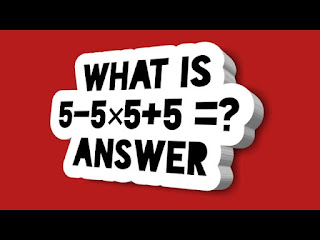0

# What's 5-5×5+5 = ? Answer# 5-5×5+5 = ?

This math quiz '5-5×5+5= ?' has gone viral on social media, many people  who tried to solve it have answers like -15, 5, 25 etc.

If you have been doubting your answer, then in this article I will solve and explain  in detail why the answer to 'What's 5-5×5+5= ?' Math Quiz is not 25. Be sure to share the Quiz to those who love Quizzes, puzzles, riddles and brain games.

I will solve Taking into consideration some Basic Mathematical rules such as :

• Order of Operation (BODMAS and PEMDAS).

• Solving from left to right.

• Signs lie behind a number. This rule is affecting many answers so you have to take it seriously and master your number line well.

## Solution: What's 5-5×5+ 5 =?

The correct answer to this tricky math quiz would be -15 not 25 or 5. Full solving and explanation below.

The solution to this question is simple but tricky. Many people always fall into the trap of not using basic mathematical principles and rules such as BODMAS and PEMDAS

BODMAS stands for:

B=Bracket,

O=Order of operation,

D=Division,

M=Multiplication,

S=Subtraction

PEMDAS stands for:

P=Parentheses,

E=Exponents,

M=Multiplication,

S=Subtraction.

So , using BODMAS,

5-5×5+5=?

First, multiply -5 × 5= - 25.

Remember in Maths a negative is attached behind a number not in front and if you multiply a negative number with a positive number your result should be negative. This is where you may get confused .

The Quiz will Now be 5 - 25 +5 = ?

Secondly 5+5 = 10.

Quiz will now be 10 - 25=? Recall that with BODMAS addition comes before subtraction and this really means you should deal with all the positive numbers in a question first whether the number comes after a negative number or not.

So, 10 - 25 = ?

-15 = ?

## Summarized solving

5-5×5+5=?

Implied 5-25+ 5 = ?

Implied 10-25= ?

Implied -15=?

Therefore, The answer to 5-5×5+5= is -15,